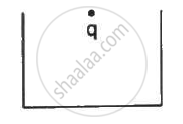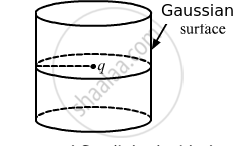Department of Pre-University Education, KarnatakaPUC Karnataka Science Class 12
Share

# A Charge Q is Placed at the Centre of the Open End of a Cylindrical Vessel (See the Figure). the Flux of the Electric Field Through the Surface of the Vessel is - Physics

ConceptElectric Flux

#### Question

A charge q is placed at the centre of the open end of a cylindrical vessel (see the figure). The flux of the electric field through the surface of the vessel is ____________ .•  zero

• q/εv

• q/2εv

• 2q/εv

#### Solution

q/2ε0
According to Gauss's Law, the flux through a closed cylindrical Gaussian surface is q/ε0. But the question is about an open cylindrical vessel. Now, take another identical vessel and make a closed Gaussian surface enclosing the charge, as shown in the following  figure.Total flux linked with the closed Gaussian surface,

Ø T =q/ε_0

Flux linked with the surface of a open ended cylindrical vessel,

Ø = (Ø"T")/2 = q/(2ε_0)

Is there an error in this question or solution?

#### APPEARS IN

HC Verma Solution for Concepts of Physics - Vol. 2 (2018 to Current)
Chapter 8: Gauss’s Law
MCQ | Q: 7 | Page no. 140

#### Video TutorialsVIEW ALL 

Solution A Charge Q is Placed at the Centre of the Open End of a Cylindrical Vessel (See the Figure). the Flux of the Electric Field Through the Surface of the Vessel is Concept: Electric Flux.
S